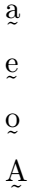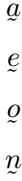# How to write tilde(~) symbol in LaTeX?

You will see very few symbols which are used a lot. But, one of them is the tilde symbol. In this tutorial, each use of the tilde symbol is represented with the help of latex.

The tiled symbol is sometimes used above the letter and sometimes below the letter and sometimes along with the letter. So, there are different commands and packages for this.

## Use tilde symbol above the letter in LaTeX

This tilde symbol is used on some special characters in spanish and Portuguese. To use the tilde symbol on a character using latex, you need to use the \~{} and \tilde{} commands.

\documentclass{article}
\begin{document}
\~{a} \; \~{n} \; \~{o}
\end{document}

Output :

ã  ñ  õ

But, you have to use \tilde{} command in math mood, you can’t use it in direct text.

\documentclass{article}
\begin{document}
$\tilde{a}$
$\tilde{n}$
$\tilde{o}$
\end{document}

Output :

ã
ñ
õ

In spanish, this symbol is used only above the letter n.

\documentclass{article}
\begin{document}
ni\~{n}o = child \\
ara$\tilde{n}$a = spider
\end{document}

Output :

niño = child
araña = spider

And in the case of Portuguese, the tilde symbol is used on the vowel a and o.

\documentclass{article}
\begin{document}
sal$\tilde{o}$es = saloons \\
coraç\~{a}o = heart
\end{document}

Output :

salões = saloons
coração = heart

It is best practice to use the latex \~{} command for spanish and Portuguese languages.

## Use tilde symbol below the letter in LaTeX

You will see very few cases where the tilde symbol has been used below the letter. In this case, we will take the help of tipa and accents packages. And tipa package contains the \textsubtilde command.

\documentclass{article}
\usepackage{tipa}
\begin{document}
\textsubtilde{a} \$6pt] \textsubtilde{e} \\[6pt] \textsubtilde{o} \\[6pt] \textsubtilde{A} \\[6pt] \end{document} Output :However, two arguments must be passed between the \underaccent command. The first is \tilde command and second is letter. \documentclass{article} \usepackage{accents} \begin{document} \[ \underaccent{\tilde}{a}$
$\underaccent{\tilde}{e}$
$\underaccent{\tilde}{o}$
$\underaccent{\tilde}{n}$
\end{document}

Output :## Use tilde symbol in mathematics

There are different parts of mathematics. Although the symbol is the same, the meaning of the symbol is not the same everywhere.

1. In Algebra, single tilde or double tiled symbol is used for approximately or approximate equal.

\documentclass{article}
\usepackage{amssymb}
\begin{document}
$\pi \sim 3.14$
$a \approx b$
% amssymb package for \thicksim and \thickapprox commands
$\pi \thicksim 3.14$
$a \thickapprox b$
\end{document}

Output :

π ∼ 3.14
a ≈ b
π ∼ 3.14
a ≈ b

Also, in the specific case, a single tiled symbol is used to subtract between two numbers.

\documentclass{article}
\begin{document}
$9 \sim 7$
$10 \sim 5$
\end{document}

Output :

9 ∼ 7 = 2
10 ∼ 5 = 5

2. In set theory, when two sets are equivalent, the equivalence of the set is denoted by a single tilde notation.

\documentclass{article}
\begin{document}
$A \sim B$
\end{document}

Output :

A ∼ B

3. In geometry, approximate symbol is used on equal symbol when two triangles are equal. For example

\documentclass{article}
\begin{document}
$ABC \sim PQR$
% \cong command for ≅ symbol
$ABC \cong PQR$
\end{document}

Output :

ABC ∼ PQR
ABC ≅ PQR

## Use tiled symbol in computer command and programming language

1. In language, bitwise complement operator and general sibling selector are denoted by tiled notation. For example

\documentclass{article}
\begin{document}
$x = \; \sim 2$
% for css general sibling selector
$div \sim p$
$div \sim h2$
\end{document}

Output :

 x = ∼ 2
div ∼ p
div ∼ h1

2. In command, if you are using a Linux operating system, you will notice that this notation indicates the home directory.

\documentclass{article}
\begin{document}
$cd \sim$
\end{document}

Output :

cd ∼#### Md Jidan Mondal

LaTeX expert with over 10 years of experience in document preparation and typesetting. Specializes in creating professional documents, reports, and presentations using LaTeX.